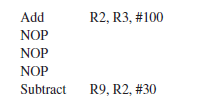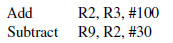# CO-Pipelining-Q6

NOP instruction is a special instruction which doesn’t perform any operation in its execution phase. Consider the following sets of instructions which are generated by a compiler.

S1:S2:Assume a 5-stage pipeline with following stages

Instruction Fetch (IF), Instruction Decode (ID), EX(Execute), Memory(MEM), and Register Write (RW).
If each stage takes 2nsec time, The time required to execute S1 minus the time required to execute S2(in nano secs). Assume that there is no data forwarding support from the hardware.asked Jun 12
reshown Jun 13

From Hamacher
WB
stage and Decode stage can not overlap when there is a data dependecy (RAW ).
With that assumption if you solve this question then answer will be 0.From Patterson

WB stage and Decode stage can overlap if there is is a data dependecy (RAW ).With this assumption if we solve the problem then answer is 2 nsec.

I feel the second approach is preferred as it optimizes the pipeline performance.

In the exam based on the answers we need to go. If the question is numerical then preffered approach is the second one.answered Jun 17 by (31,090 points)
0 is correct oneanswered Jun 13 by (19,690 points)
can you share the solution
Please provide explanation. How come answer is zero?
the answer should be 2

S1 can be executed like

 IF ID EX M WB - - - IF ID EX M WB

So total 9 clock cycle.

S2 will be executed like

 IF ID EX M WB --- --- IF ID EX M WB

Here S2 take 8 clock cycle. So difference of 1clock cycle i.e 2nsec.

Here also no data forwarding is used. Only it is taken that WB stage and ID stage is happening at a time. In the 1st half of the clock cylce WB and then in the 2nd half ID (as taught in the lectures.)

So why answer is 0 i.e WB and ID are happening in two different stages. @gatebook

answered Jun 14 by (330 points)
I think you are right
first will take 9 clock cycles right in second it will take 9 clock cycles as data forwarding is not supported therefore answer comes out to be zero ID and WB can not occur within same cycle as data forwarding is not supported so zero is correct answer
@surbhipro19 please check what is forwarding here http://web.cs.iastate.edu/~prabhu/Tutorial/PIPELINE/forward.html
Sudipta, check my answer.answered Jun 17 by (6,590 points)